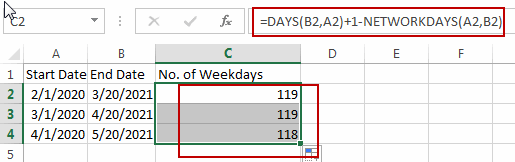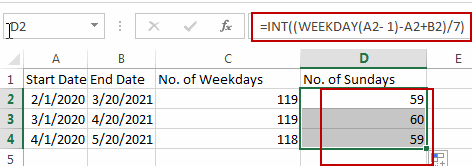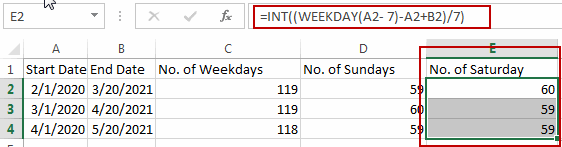# How to Calculate Number of Weekends between Two Dates in Excel

This post will guide you how to count the number of weekends between two given dates in Excel. How to find out how many weekends are between two dates in Excel. How to count the number of Sundays between two dates with a formula in Excel.

## Calculate the Number of Weekdays between Two Dates

Assuming that you have a list of data in range A1:B4, in which contain start dates and end dates. And you want to calculate the number of weekend days between start date and end date. How to do it. You can use a formula based on the NETWORKDAYS function and the DAYs function to calculate the number of weekends between two given dates.

Here is the formula that it will count the total number of weekend days between two given dates:

`=DAYS(B2,A2)+1-NETWORKDAYS(A2,B2)`

Type this formula into cell C2, and press Enter key on your keyboard, and then copy this formula from cell C2 to range C3:C4 to apply this formula.Let’s see how this formula works:

The NETWORKDAYS function try to calculate the number of working days (weekdays) between two dates, and the DAYS function will count the number of days between two dates. So you can use days number to subtract working days, then you will get the number of weekends days.

## Calculate the Number of Sundays between Two Dates

If you only want to count the number of Sundays between two given dates in Excel, you can use an Excel formula based on the INT function and the WEEKDAY function. Like this:

`=INT((WEEKDAY(B1- 1)-A2+B2)/7)`

Type this formula into cell D2, and press Enter key on your keyboard.Note: if you want to count the number of other week numbers (1-7, 1 is Sunday, 2 is Monday, 3 is Tuesday, 4 is Wednesday, 5 is Thursday, 6 is Friday, 7 is Saturday ) between two dates, such as: Saturday, you just need to replace the number 1 with 7 in the above formula. Like this:

`=INT((WEEKDAY(B1- 7)-A2+B2)/7)`### Related Functions

• Excel INT function
The Excel INT function returns the integer portion of a given number. And it will rounds a given number down to the nearest integer.The syntax of the INT function is as below:= INT (number)…
• Excel WEEKDAY function
The Excel WEEKDAY function returns a integer value representing the day fo the week for a given Excel date and the value is range from 1 to 7.The syntax of the WEEKDAY function is as below:=WEEKDAY (serial_number,[return_type])…
• Excel DAYS function
The Excel DAYS function returns the number of days between two dates.The syntax of the DAYS function is as below:= DAYS (end_date,start_date)…

Related Posts

How to Count Dates by Day of Week in Excel

This post will guide you how to count days of week in the range of dates using a formula in Excel 2013/2016 or Excel office 365. How do I count dates by day of week in Excel. Count Dates by ...

How to Sum Data by Weekday with Different Formulas/Functions in Excel

To generate a business summary report for further investigation, we can sum income or profits for a date like Monday, Tuesday …etc. to check on which date we have the top income in a week, then we can estimate the ...

How to Highlight Cell or Row If Date Is In Current Day/Week/Month in Excel

This post will guide you how to highlight cell if date is the current day or is in the current week or month in Excel. How do I highlight row if date is in current week or month with conditional ...

Filter Out Weekends and Weekday

This post will guide you how to filter out weekend or weekday from a list of data in Excel. How do I filter out the data in all Weekends in Excel. How to filter out all data in all weekdays ...

Determine If a Date Falls on the Weekend

This post will guide you how to determine if a date falls on the weekend in Excel. How do I check if a date falls on the weekend with a formula in Excel. How to check if a date is ...

Sidebar